A Stochastic Programming Based Scheduling and Dispatch for Smart Grid with Intermittent Wind Power | OMICS International
Global Journal of Technology and Optimization
All submissions of the EM system will be redirected to Online Manuscript Submission System. Authors are requested to submit articles directly to Online Manuscript Submission System of respective journal.

# A Stochastic Programming Based Scheduling and Dispatch for Smart Grid with Intermittent Wind Power

Mingsheng Gao*, Jian L, Wei Li and Xiao Yao

College of Internet of Things Engineering, Hohai University, China

Corresponding Author:
Gao M
College of Internet of Things Engineering
Hohai University, China
Tel: +86-25-8378 7955
E-mail: [email protected]

Received Date: December 26, 2015; Accepted Date: January 27, 2016; Published Date: January 29, 2016

Citation:Gao M, Jian L, Li W, Yao X (2016) A Stochastic Programming Based Scheduling and Dispatch for Smart Grid with Intermittent Wind Power. Global J Technol Optim 7:191. doi:10.4172/2229-8711.1000191

Copyright: © 2016 Gao M, et al. This is an open-access article distributed under the terms of the Creative Commons Attribution License, which permits unrestricted use, distribution, and reproduction in any medium, provided the original author and source are credited.

Visit for more related articles at Global Journal of Technology and Optimization

#### Abstract

From electricity supply side, power providers’ electricity energy is assumed to comprise two parts: one is procured from the wholesale market/national power grid, and the other is produced by their own wind generations. From electricity demand side, we assume two categories of energy users, namely traditional energy users and opportunistic energy users. Taking a unit time into account, this paper models the profits of power providers as a stochastic programming problem with three system parameters to be determined, i.e., the electricity procurement, the day-ahead price corresponding to traditional energy users and the real-time price corresponding to opportunistic energy users. Our objective is to maximize providers’ profits while preserving the balance between electricity supply and demand. To solve this problem, we first convert the stochastic programming problem into a nonlinear programming problem with constraint conditions; we then solve it using standard nonlinear optimization methods. With our proposed model, power provider can not only maximize the profits, but also can easily achieve the tradeoff between the profits of the power providers and the penetration of wind energy by tuning the system parameters. Numerical results show our proposed method can potentially benefit both power providers and energy users.

#### Keywords

Dispatch; Smart grid; Stochastic programming; Wind generation

#### Introduction

Motivation

The growing interest in the design of smart grids is driven by the desire to integrate volatile renewable energy resources, such as wind, solar, bio-fuel, and geothermal energy, into existing power systems [1- 5]. In the past decades, wind energy has received more attention than other renewable sources. This is due to the fact that, after the initial land and capital costs, there is essentially no cost involved in the production of power; moreover, the wind energy is generally reckoned to be environmentally friendlier than the impacts of thermal energy sources . For modern power systems with wind generation, the challenges, to a large extent, originate from the uncertainties both on the supply side and the demand side. On the supply side, wind generation is generally perceived to be stochastic, volatile and uncontrollable. Therefore, how to efficiently and reliably integrate the intermittent renewable resources into existing power systems is of importance. To efficiently predict the output of the intermittent wind generation; there have been extensive studies so far [7-10]. On the demand side, the uncertainty is induced by a class of energy users, called opportunistic energy users. Basically, opportunistic energy users have a desire to optimize their electricity consumption profiles, aiming to minimize their electricity costs. Different from traditional energy users who are generally not sensitive to the price of electricity but the reliability of the supply of electricity, opportunistic energy users are very sensitive to the real-time retail price of electricity; they access the power system in an opportunistic manner according to the real-time pricing received from electrical power providers or retailers: when the real-time retail price is beyond their price acceptance levels, there are probably a large number of opportunistic energy users accessing the power system; conversely, there might be fewer opportunistic energy users accessing the power system. Out of question, this imposes additional difficulties on system operators in maintaining additional generation and reserves. From the viewpoint of power generation plants, fully meeting the demand of electricity while minimizing the cost is their major concern. Here the cost consists of the economic cost and environmental cost. The economic cost includes the cost of procuring electricity from the wholesale electricity market, and the cost of starting-up generating units in case the electricity supply cannot meet the demand of electricity; while the environmental cost mainly involves the cost of CO2 emissions. To this end, power generation plants are expected to enhance the penetration of wind energy as high as possible. In this paper, we focus on the dispatch problem of power systems with intermittent renewable resources (e.g. wind generation), addressing the following challenges: 1) the supply uncertainty as a result of the volatility and nonstationarity of wind generation, and 2) the demand uncertainty due to a large number of opportunistic energy users and their stochastic behaviors. Our objective is to incorporate wind generation into the classical economic dispatch problem and to find out its optimal solutions.

Related work

There have been lots of approaches that were developed to address the economic dispatch problem in the past decade. For example, in  the authors proposed a statistical interpolation method, aiming to generate a time series of system-aggregated wind power production. By making use of model predictive control (MPC) method,  developed a multi-objective dispatch model by treating future wind generation in the scheduling horizon as a dynamic process specified by an ARMA model, with the objective of minimizing both generation and environmental costs simultaneously. With the considerations of cost, reliability, and environment,  investigated the impacts of high integration of wind power on system operation. In  a direct search based iterative algorithm was proposed to obtain the optimal wind- thermal coordination dispatch in an isolated power system. To solve the stochastic economic dispatch problem, the authors in  developed a comprehensive method. In  the authors developed a multi-objective optimization model by integrating the environmental consideration into the method . In , a genetic algorithm (GA) was proposed to coordinate the wind and thermal generation dispatch. In , cost function merger (CFM) method was adopted to solve the stochastic generation cost problem. In  the effects of wind energy produced by independent power producers (IPP) in an electricity market on the generation dispatch were addressed. In  a risk-limiting dispatch in contrast to worst-case dispatch for traditional generation planning was proposed by treating scheduling with known demand and uncertain supply as a multi-stage decision problem with recourse.

Our contribution

The main contributions of this paper can be summarized as follows:

1. We propose a stochastic programming model for the dispatch problem one period of time by accounting for the two types of uncertainties, namely the uncertainty of energy users and that of the supply of wind energy, and the confidence level of the penetration of wind generation.

2. We demonstrate in detail how to solve this stochastic programming to determine the system parameters, i.e., the dayahead pricing, the real-time pricing and the day-ahead procurement of electricity energy. Specifically, we first transform the stochastic programming into a nonlinear programming, after which we find the optimal solutions using standard convex optimization methods.

3. The tradeoff between the profit margin and the penetration of wind generation can be easily achieved by adjusting the realtime pricing and/or the day-ahead pricing given the volatility and intermittency of wind energy.

Paper outline

The paper is organized as follow. In section 4 we present a system model with uncertain wind generation and energy users. In section 5 we conduct an analysis on statics of wind power. We formulate the dispatch problem and solve the stochastic programming problem in section 6. In section 7, we present a discussion of the numerical results achieved when several different wind and cost scenarios are applied to the model. Finally, we conclude this paper and suggest future work in section 8.

#### System Model

In our model, we assume that there is a power generation plant (or an electricity retailer) serving an uncertain number of energy users. It owns some wind-powered generators by itself. To provide the supply of electricity at one period of time (called unit time), it first estimates the wind energy probably generated and the total number of energy required during the unit time, then procures electricity from the electricity wholesale market (or national power grids). Finally, it announces the day-ahead retail price for traditional energy users and the real-time retail price for opportunistic energy users at proper instants, respectively, with the objective of maximizing its profit margin while balancing the electricity supply and demand. To understand in depth our dispatch model, it is necessary for us to describe in detail the uncertainties and the pricing mechanism in the smart grid with intermittent wind energy. The notations used in this paper are listed in Table 1.

Notation Description
c2 Real-time retail price for opportunistic   users
c Price acceptance threshold for traditional   users
ca Wholesale price in the  wholesale market cp
e0 Per-unit  energy  consumption for one opportunistic user
λ Arrival rate of a Poisson distribution for opportunistic users
θ Conﬁdence level about the relationship between energy supply and    demand
W Energy  supply of  wind generation
fW(w) Probability density function (pdf) of random variable W
FW (w) Cumulative distribution function  (cdf)  of  random variable W ξ
hi Probability of the event that the number of the traditional users is i
pj Probability of the event that the number of the opportunistic users is j V
fV(v) Probability density function (pdf) of random variable V
FV (v) Cumulative distribution function (cdf) of random   variable V vi
vr Rated wind speed
vo Cut-out  wind speed
c Scale factor  of theWeibulldistribution
k Shape factor of the  Weibulldistribution
wr Rated windpower

Table 1: Notations used in the dispatch algorithm.

Supply of energy sources and costs

For a power generation plant, electricity resource is made up of three parts: the first is the procurement from electricity wholesale market (or national power grids); the second is generated by the power generation plant itself through the combustion of fossil fuels such as coal and oil; and the last can be generated from the intermittent renewable resources (i.e. wind generation). The first part is the main energy source of the power generation plant. This is because electricity wholesale markets (or national power grids) own the majority of electricity resources where the up-to-the-minute relationship between electricity supply and electricity demand can be explicitly reflected. This implies the wholesale price ca is usually lower than the cost for which the power generation plant starts up its own generation units (e.g., gas turbines). While for the second part, it is induced only if the total supply (i.e. the sum of the procurement of electricity and the intermittent wind energy) is less than the total demand. In this case, the power generation plant has to start up its own generators to meet the demand of electricity, which leads to a higher generation cost. In this paper, we refer to this cost as the penalty price cp. Typically, Cp is much higher than ca. For the third part (i.e., wind generation), there is essentially no cost involved in the production of power after the initial land and capital costs since we have assumed the power generation plant owns the wind-powered generators itself. It is well known that wind generation is, to a large extent, influenced by wind speed, which is a random variable following the Weibull distribution [6-12]. Its statistical properties will be in detail analyzed Section III. From the above discussion, it suggests that for the power generation plant, it should strive to achieve the balance between supply and demand. This is because if the supply is larger than the demand, some of energy will be wasted; however, a penalty price will be imposed on the power generation plant if the electricity procurement together with the wind energy cannot satisfy the demand.

Pricing for various types of energy users

The pricing is concerned with two types of energy users, namely the traditional energy users and the opportunistic energy users. Basically, the traditional energy users are in advance informed of the day-ahead retail price (c1) corresponding to one specific time unit. By contrast, whether opportunistic energy users access the power system or not thoroughly depend on the real-time retail price (c2), which is based on the availability of the procurement (s) and the renewable resource. Intuitively, the real-time retail price is expected to be as low as possible so that opportunistic energy users can minimize their electricity costs. It is worth pointing out that risk may occur when the amount of wind generation is small; c2 might be higher than ca, which will be discussed in Section V.

Demand of energy sources and profits

Based on , the energy demand of traditional energy users Dc in a slot could be expressed as Dc = ψ · ec where ψ is a Poisson random variable with mean λc .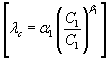(1)

Where α1 is an empirical coefficient, C1 is a price acceptance level for the traditional energy users, and β1 is the price elasticity of the traditional energy users, satisfying -1 ≤ β1 < 0 . To describe the demand of opportunistic energy users, we make the following assumptions: i) opportunistic energy users arrive following a Poisson process with rate λ, which is constant within one time unit; and ii) in each time unit, each opportunistic user arriving in the power system decides to accept or reject the announced real-time price C2 by comparing with a price acceptance level2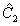. This price acceptance level is randomly chosen and is i.i.d across the opportunistic users. Based on this, we assume the total energy demand from opportunistic energy users is given by Do = ξ • eo, where ξ is a Poisson random variable with mean λo: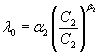(2)

Here α2 is also an empirical coefficient and β2 is the price elasticity of the traditional energy users, satisfying β2 < -1 . It is important to note that, in our model we assume there will be enough energy users accessing the power system if the retail price(s) (either c1 or c2 , or both) is/are low enough (lower than their price acceptance level). Define Δ = Dc + Do - S. Clearly, it is reasonable that we assume Δ ≥ 0 throughout this paper. From the aforementioned discussion, it is seen that our dispatch model can be easily extended to multiple timescale (e.g., one timescale corresponds to one unit time).

#### The Distribution of Wind Power

Typically, the wind power is a function of wind speed V, which is a random variable. For short-term (say in hour) the mean wind speed V follows the Weibull distribution; however, for long-term (say in month or year) the mean wind speed V can be expressed by a Normal distribution function . In this paper we address the dispatch problem in one unit time (in hour). As such, the speed V in one unit time can be described by the Weibull distribution. The cdf of the Weibull distribution takes the following form: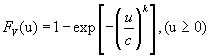(3)

Where c > 0 and k > 0 are respectively referred to as the scale factor and shape factor.

Correspondingly, the pdf of V is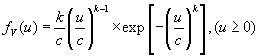(4)

Figure 1 shows the Weibull probability density function f (u) for different shape factor ks. The relationship between the input wind power and the output electric power (recall that the latter has been briefly referred to as wind power in the foregoing section) relies on several factors, such as the efficiencies of generator, wind rotor, gearbox, and inverter, depending on what kind of turbine under investigated. For a generic wind turbine, some researchers used a simplified model to characterize the relation between the wind power and wind speed: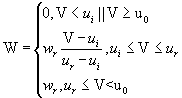(5)

Figure 2 shows the curve related to wind output power versus wind speed.

Define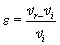. By the probability theory, we can obtain the pdf of W as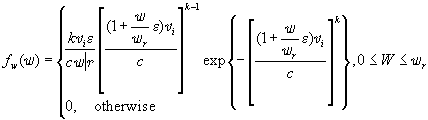(6)

According to (5), the cdf of W yields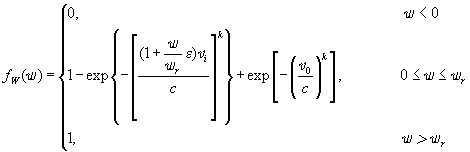(7)

#### Problem Formulation and Its Solutions

In smart grids, renewable resources (e.g. wind energy, solar energy) are expected to be exploited as much as possible. To this end, a certain utilization rate of wind generation, which is defined as the ratio of the wind generation used to the wind generation generated, should be guaranteed at the power generation sides.

Let Δ Dc+Do-s Throughput this paper we assume the procurement of energy for one power generation plant cannot meet the total demand of energy. That is to say, Δ > 0. Basically, there are two cases: 1) if Δ > W, then all the wind generations will be used; that is, the utilization rate of wind generation is 100%, and therefore extra power energy is still required to meet the total demand of energy, which results in a penalty cost cp (Δ−W); and 2) if Δ ≤ W, then no extra power energy is required. In case 2), we assume Dc + Do - s ≥ θ so as to guarantee a certain utilization rate of wind generation. Putting the aforementioned discussion in the format of an optimization problem, the mathematical model can be formulated as a stochastic programming problem: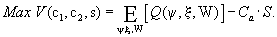(8a)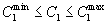(8b)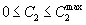(8c)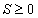(8d)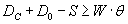(8e)

Where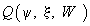is given by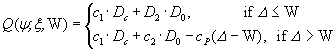(9)

Due to the mutual independence among random variables ψ, ξ and W, it follows that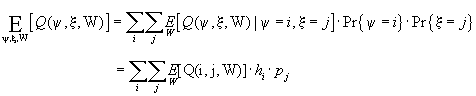(10)

Thus, the stochastic programming problem (8a)-(8e) can be further rewritten as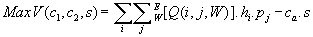(11a)

Subject to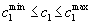(11b)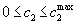(11c)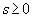(11d)

Let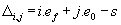. It follows from (8e) and (9) that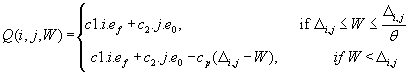(12)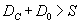(13)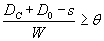(14)

To solve the stochastic programming problem (11a)-(11d), we next compute. Let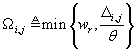,then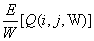can be calculated as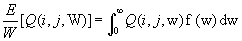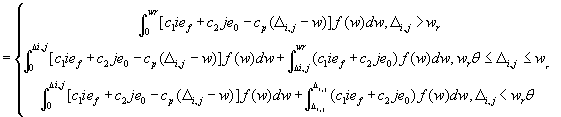(15)

To simplify (15), we now calculate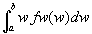where a, b ∈ [vi, vr]. By (6), it follows that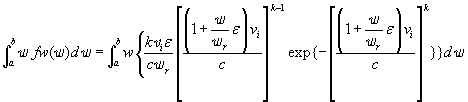Let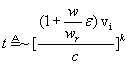, then the above equation can be expressed as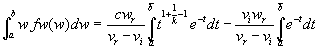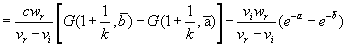(16)

Where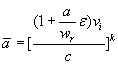and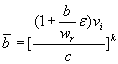, and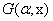is the incomplete gamma function, which is defined as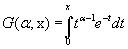Using (7) and (16), (15) can be rewritten as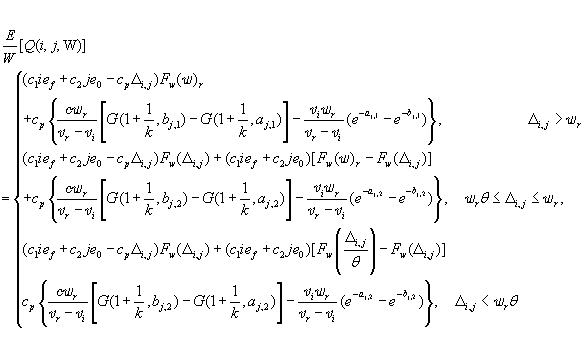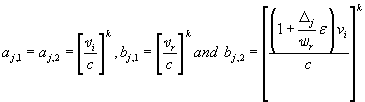.

Substituting (17) into (11a), then the stochastic programming problem (11a)-(11d) is converted to a nonlinear programming problem with constraint conditions, which can be solved via standard convex optimization methods.

#### Numerical Examples

To verify the concreteness, we now study, via numerical experiments, the performance of the proposed dispatch algorithm. Our focus is mainly on the impact of some crucial parameters which can be adjusted by energy suppliers on the dispatch algorithm.

Determination of the weibull factors c and k

As shown in Figure 1, the selection of the scale factor c and the shape factor k of the Weibull distribution has a significant impact on the Weibull pdf fV (v). For a specific power generation plant, the local wind speed distribution is generally deterministic. Without loss of generality, the wind speed nearby the power generation plant is assumed to range from v1 m/s to v2 m/s. Next we shall determine the Weibull Factors c and k.

According to (3),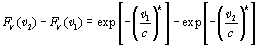(18)

Define φ(c, k) = FV (v2) − FV (v1). Then we have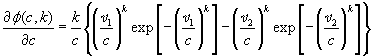(19)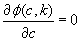For a given k, we can derive the optimal copt as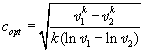(20)

In this section, we conduct the numerical experiments using the default parameters, which are listed in Table 2, unless specified otherwise. It is important to note that the stochastic programming problem (11a)-(11d) is a concave maximization problem, and that it is impossible to obtain the closed-form solutions of this problem. In this paper we solve this problem using the Interior Point Method .

Parameter Value Unit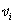5 m/s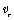15 m/s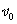30 m/s
α1 0.5
β1 -1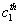1 \$/KWh
α2 100
β2 -2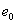0.001 KWh
c 10
k 2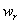1 MVA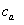0.1 \$/KWh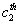0.2 \$/KWh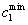0.15 \$/KWh0.25 \$/KWh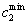0.01 \$/KWh0.3 \$/KWh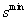0 KWh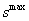2 KWh
Cp 0.4 \$/KWh
θ 0.8

Table 2: Parameter used in numerical result.

The Optimal Solutions under different scenarios

Impact of the wholesale price ca: Figure 3 illustrates the optimal solutions for Ca = 0.1 and 0.2 as cp varies from ca to 5ca. From Figure 3a we can observe that 1) c1 and c2 for ca = 0.2 are lower than their counterparts for ca = 0.1; 2) when cp is large, c2 is lower than c1, while the boot is on the other foot when cp is small. For example, when cp ≤ 2ca, c1 > c2, while c1 < c2 when cp > 2ca. Figure 3b shows the optimal procurement of electricity s for ca = 0.1 is almost the same as that for ca = 0.2. Figure 3c suggests that the optimal benefit margin for ca= 0.2 is larger than that for ca = 0.1. This can be explained as follows. Given the same distribution of wind generation, the smaller the amount of the procurement of electricity, the smaller the cost for the electricity power plant to undertake, thus resulting in a higher profit margin.

Impact of the Parameter c1max: Figure 4 shows the optimal solutions as a function of cp for c1max = 2 and 2.5, respectively. As shown in Figure 4a and c2 is larger than c1 for each case as cp increases. On the other hand, the optimal procurement of electricity s for c1 max = 0.25 is larger than that forc1 max = 0.2, as shown in Figure 4b. Accordingly, a smaller s corresponds to a larger benefit margin, as shown in Figure 4c. The utilization rates of wind generation are shown in Figure 4d for c1 max = 0.2 and 0.25, respectively. By comparing with Figure 4c, we can see that when c1 max is lowered, more traditional energy users will access the power grid, leading to a high procurement of electricity. However, this also introduces a higher penetration of wind generation. The decrease of the profit margin of the electricity power plant is generally assumed to be compensated by the less emission of carbon dioxide, which is beyond the scope of this paper.

Impact of the Parameter c2 max : The conclusions in Figure 5 still hold as those in Figure 4 when c2 max = 0.15 and 0.3 respectively. It is seen that a small c2 max motivates more opportunistic energy users to access the power system, enhancing the penetration of wind generation despite the decreasing of the profit margin, as shown in Figure 5d.

Impact of the parameter wr: In Figure 6 we evaluate the impact of the rated wind power wr on the optimal solutions when wr = 0.5 MVA and 1 MVA, respectively. From Figures 6a -6c, one can make the following remarks. First, with the increase of cp, c2 increases (Figure 6a). Second, a larger wr leads to a smallers (Figure 6b). This is straightforward because a larger wr implies a larger supply of electricity. Third, a larger wr corresponds to a larger profit margin (Figure 6c). This is due to the fact that a smaller s decreases the procurement cost of the electricity power plant, thus resulting in a higher profit margin.

#### Conclusion

In this paper, we present an economic dispatch model that integrates wind generations into existing power grids. Wind energy is modeled as a Gaussian distribution. By day-ahead scheduling and real-time scheduling, we investigate timescale-based dispatch and scheduling for smart grids, with the objective of combating the variability and uncertainty of the renewable resource. More specifically, in day-ahead scheduling, with the statistical information on wind generation and energy demands, we characterize the optimal procurement of the energy supply and the day- ahead retail price for the traditional energy users; in real-time scheduling, with the realization of wind generations and the load of traditional energy users, we optimize real-time prices to manage the opportunistic energy users so as to achieve system-wide reliability. The proposed dispatch model is formulated by a stochastic programming problem. Then the optimization problem is numerically solved. Numerical results show that the optimal solutions of the stochastic economic dispatch problem are sensitive to price acceptance threshold, the wholesale Price and Confidence Level. Our future work will focus on designing more precise dispatch and scheduling algorithms so as to reflect practical power systems.

#### References

Select your language of interest to view the total content in your interested language

### Article Usage

• Total views: 9291
• [From(publication date):
April-2016 - Nov 19, 2019]
• Breakdown by view type
• HTML page views : 9086Can't read the image? click here to refresh